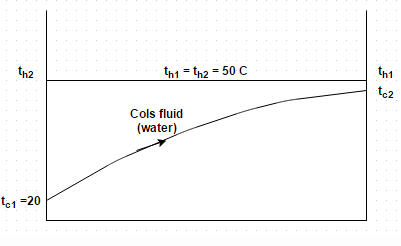Test: Heat Exchanger Effectiveness

# Test: Heat Exchanger Effectiveness

Test Description

## 10 Questions MCQ Test Heat Transfer | Test: Heat Exchanger Effectiveness

Test: Heat Exchanger Effectiveness for Chemical Engineering 2022 is part of Heat Transfer preparation. The Test: Heat Exchanger Effectiveness questions and answers have been prepared according to the Chemical Engineering exam syllabus.The Test: Heat Exchanger Effectiveness MCQs are made for Chemical Engineering 2022 Exam. Find important definitions, questions, notes, meanings, examples, exercises, MCQs and online tests for Test: Heat Exchanger Effectiveness below.
Solutions of Test: Heat Exchanger Effectiveness questions in English are available as part of our Heat Transfer for Chemical Engineering & Test: Heat Exchanger Effectiveness solutions in Hindi for Heat Transfer course. Download more important topics, notes, lectures and mock test series for Chemical Engineering Exam by signing up for free. Attempt Test: Heat Exchanger Effectiveness | 10 questions in 10 minutes | Mock test for Chemical Engineering preparation | Free important questions MCQ to study Heat Transfer for Chemical Engineering Exam | Download free PDF with solutions
 1 Crore+ students have signed up on EduRev. Have you?
Test: Heat Exchanger Effectiveness - Question 1

### Capacity ratio is defined as the product of

Detailed Solution for Test: Heat Exchanger Effectiveness - Question 1

The product mass and specific heat of a fluid flowing in a heat exchanger is known a s capacity ratio.

Test: Heat Exchanger Effectiveness - Question 2

### A single pass shell and tube heat exchanger, consisting of a bundle of 100 tubes (inner diameter 25 mm and thickness 2 mm) is used for heating 28 kg/s of water from 25 degree Celsius to 75 degree Celsius with the help of a steam condensing at atmospheric pressure on the shell side with condensing heat transfer coefficient 5000 W/m2 degree. Make calculation for overall heat transfer coefficient based on the inner area. Take fouling factor on the water side to be 0.002 m2 degree/W per tube and neglect effect of fouling factor on the shell side and thermal resistance of the tube wallDetailed Solution for Test: Heat Exchanger Effectiveness - Question 2

Q = m (t c2 – t c1). Re = 7394, Pr = 3.53 and Nu = 47.41. I/U = I/h + R + r i/(r 0) (h 0).

Test: Heat Exchanger Effectiveness - Question 3

### Which of the following is not associated with heat exchanger?

Detailed Solution for Test: Heat Exchanger Effectiveness - Question 3

The correction factor i.e. Mc Adam’s is associated with laminar film condensation on a vertical plate.

Test: Heat Exchanger Effectiveness - Question 4

The engine oil at 150 degree Celsius is cooled to 80 degree Celsius in a parallel flow heat exchanger by water entering at 25 degree Celsius and leaving at 60 degree Celsius. Estimate the exchanger effectiveness

Detailed Solution for Test: Heat Exchanger Effectiveness - Question 4

Effectiveness = (t h 1 – t h 2) C h /C MIN (t h 1 – t c 2).

Test: Heat Exchanger Effectiveness - Question 5

Consider the above problem, if the fluid flow rates and the inlet conditions remain unchanged, workout the lowest temperature to which the oil may be cooled by increasing length of the exchanger

Detailed Solution for Test: Heat Exchanger Effectiveness - Question 5

Effectiveness = 1 – [exponential [- NTU (1 – C)]/1 + C].

Test: Heat Exchanger Effectiveness - Question 6

In a surface condenser, the water flowing through a series of tubes at the rate of 200 kg/hr is heated from 15 degree Celsius to 75 degree Celsius. The steam condenses on the outside surface of tubes at atmospheric pressure and the overall heat transfer coefficient is estimated at 860 k J/m2 hr degree. Find the effectiveness of the heat exchanger. At the condensing pressure, stream has saturation temperature 0f 100 degree Celsius and the latent heat of vaporization is 2160 k J/kg. Further, the steam is initially just saturated and the condensate leaves the exchanger without sub-cooling i.e. only latent heat of condensing steam is transferred to water. Take specific heat of water as 4 k J/kg K

Detailed Solution for Test: Heat Exchanger Effectiveness - Question 6

Effectiveness = 1 – exponential (- NTU) and Effectiveness = C (t h 1 – t h 2)/C MIN (t h 1 – t c 2).

Test: Heat Exchanger Effectiveness - Question 7

Consider the above problem, find the tube length. Let the diameter of tube is 25 mm

Detailed Solution for Test: Heat Exchanger Effectiveness - Question 7

NTU = U (π d l)/C.

Test: Heat Exchanger Effectiveness - Question 8

For evaporators and condensers, for the given conditions, the logarithmic mean temperature difference for parallel flow is

Detailed Solution for Test: Heat Exchanger Effectiveness - Question 8

The temperature of one of the fluid remains constant during the flow passage.

Test: Heat Exchanger Effectiveness - Question 9

Water (specific heat = 4 k J/kg K) enters a cross flow exchanger (both fluids unmixed) at 15 degree Celsius and flows at the rate of 7.5 kg/s. It cools air (C = 1 k J/kg K) flowing at the rate of 10 kg/s from an inlet temperature of 120 degree Celsius. For an overall heat transfer coefficient of 780 k J/m2 hr degree and the surface area is 240 m2, determine the NTU

Detailed Solution for Test: Heat Exchanger Effectiveness - Question 9

NTU = U A/C MIN = 5.2.

Test: Heat Exchanger Effectiveness - Question 10

Consider the above problem, find the capacity ratio of the heat exchanger

Detailed Solution for Test: Heat Exchanger Effectiveness - Question 10

Capacity ratio = 10/30 = 0.333.

## Heat Transfer

58 videos|70 docs|85 tests
 Use Code STAYHOME200 and get INR 200 additional OFF Use Coupon Code
Information about Test: Heat Exchanger Effectiveness Page
In this test you can find the Exam questions for Test: Heat Exchanger Effectiveness solved & explained in the simplest way possible. Besides giving Questions and answers for Test: Heat Exchanger Effectiveness, EduRev gives you an ample number of Online tests for practice

## Heat Transfer

58 videos|70 docs|85 tests